# Selina Solutions Concise Maths Class 10 Chapter 9 Matrices Exercise 9(D)

This is the last exercise of the chapter and it combines the problems of all the important topics discussed in the chapter. Students wanting to strengthen their fundamentals and attain a good grip over the subject should use the Selina Solutions for Class 10 Maths. The solutions are prepared in a simple language according to the latest ICSE marking schemes. The solutions to the Concise Selina Solutions for Class 10 Maths Chapter 9 Matrices Exercise 9(D) can be effortlessly downloaded in PDF format from the links given below.

## Selina Solutions Concise Maths Class 10 Chapter 9 Matrices Exercise 9(D) Download PDF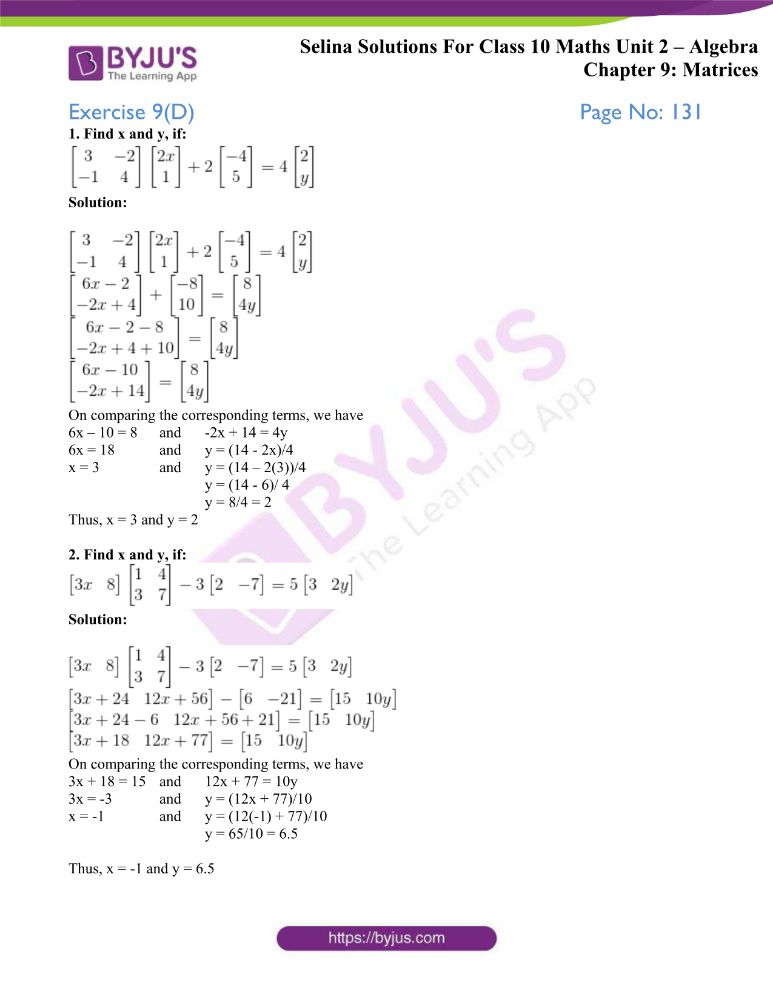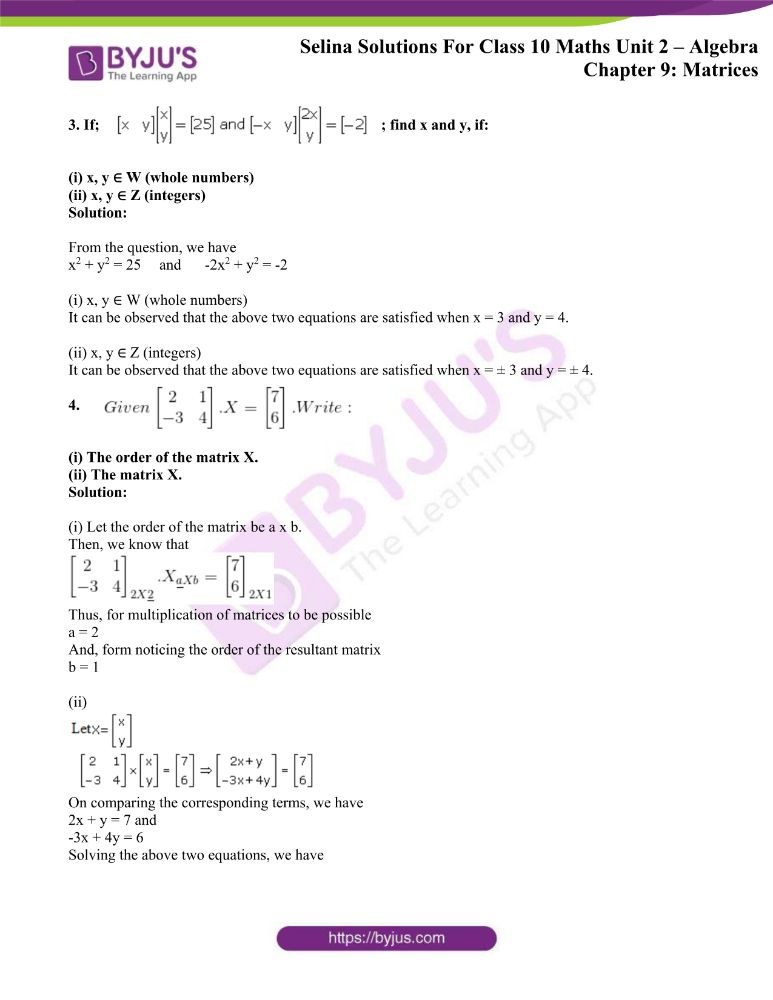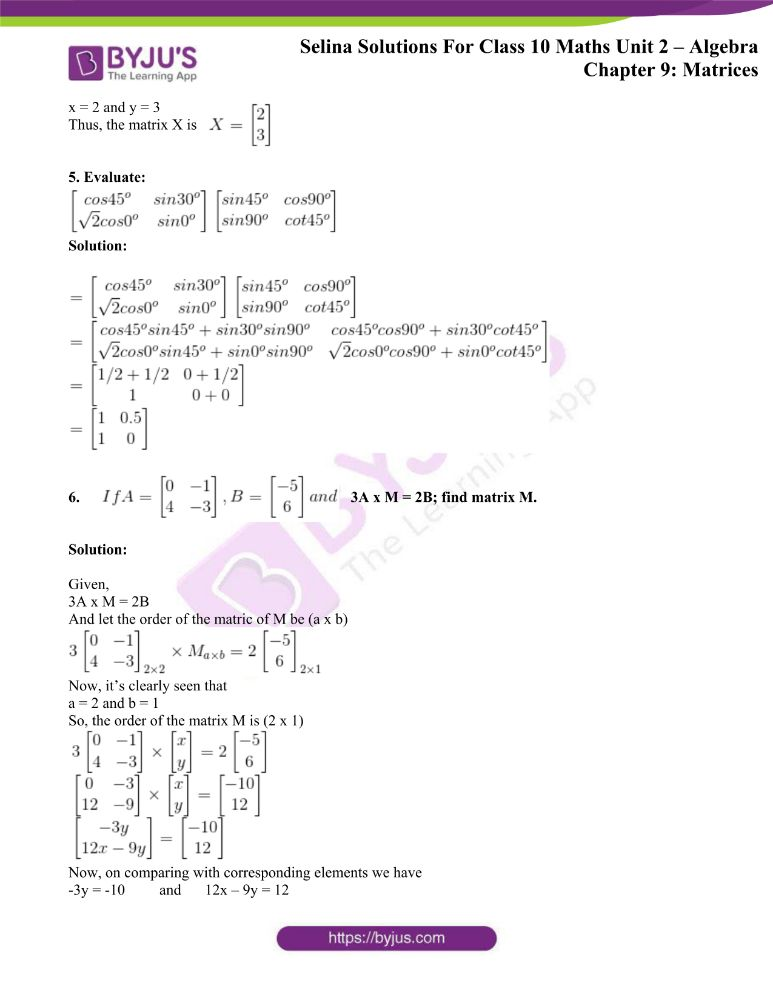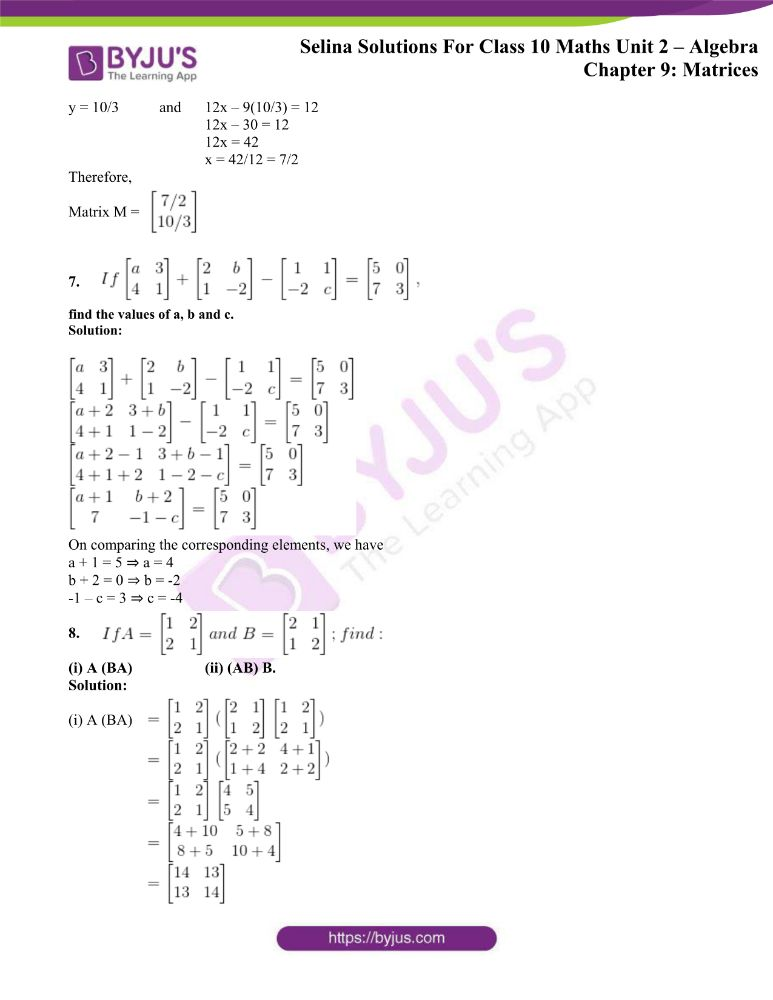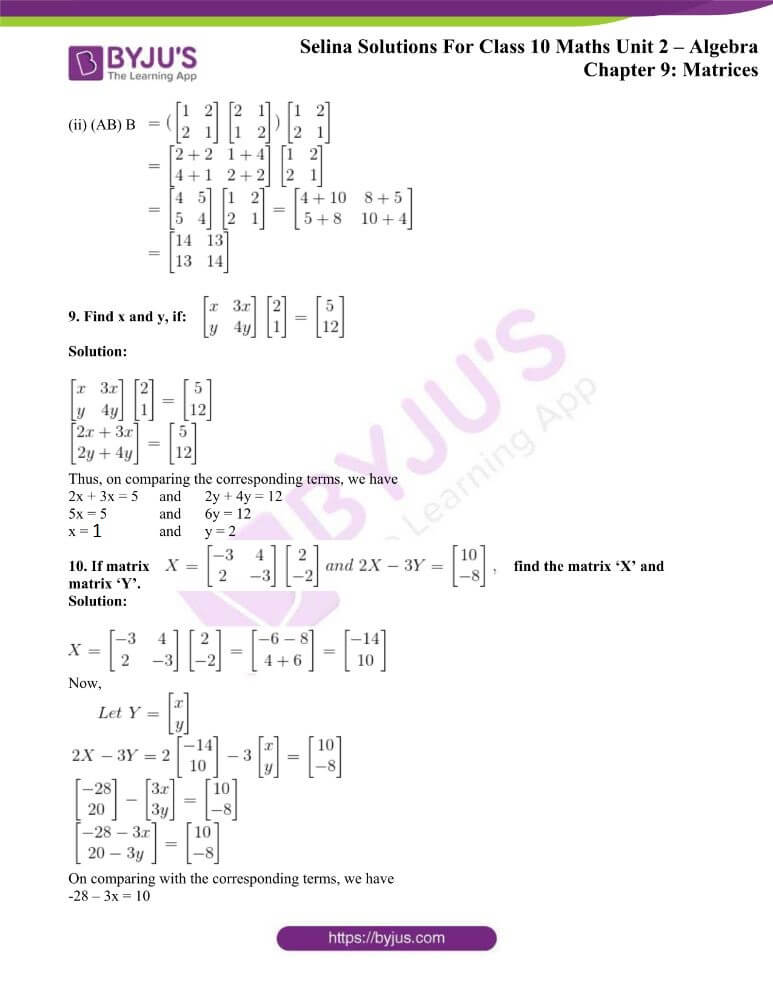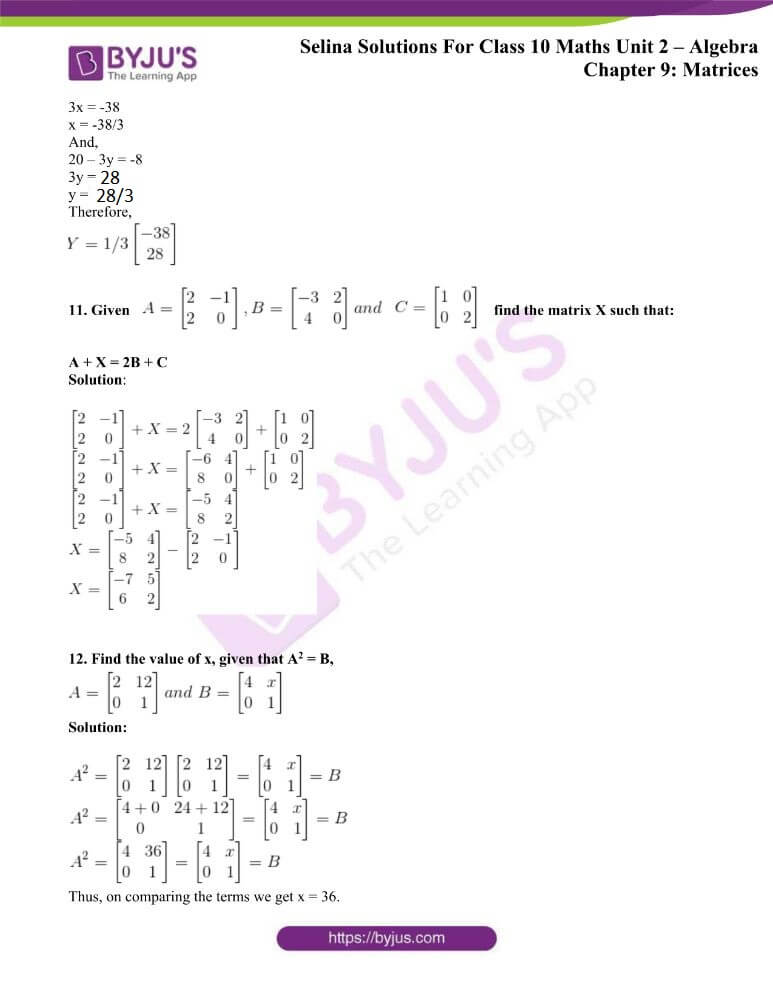### Access other exercises of Selina Solutions Concise Maths Class 10 Chapter 9 Matrices

Exercise 9(A) Solutions

Exercise 9(B) Solutions

Exercise 9(C) Solutions

### Access Selina Solutions Concise Maths Class 10 Chapter 9 Matrices Exercise 9(D)

1. Find x and y, if: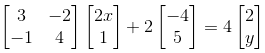Solution: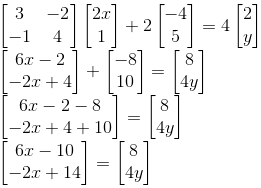On comparing the corresponding terms, we have

6x – 10 = 8 and -2x + 14 = 4y

6x = 18 and y = (14 – 2x)/4

x = 3 and y = (14 – 2(3))/4

y = (14 – 6)/ 4

y = 8/4 = 2

Thus, x = 3 and y = 2

2. Find x and y, if: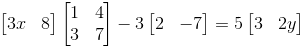Solution:On comparing the corresponding terms, we have

3x + 18 = 15 and 12x + 77 = 10y

3x = -3 and y = (12x + 77)/10

x = -1 and y = (12(-1) + 77)/10

y = 65/10 = 6.5

Thus, x = -1 and y = 6.5

3. If;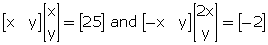; find x and y, if:

(i) x, y ∈ W (whole numbers)

(ii) x, y ∈ Z (integers)

Solution:

From the question, we have

x2 + y2 = 25 and -2x2 + y2 = -2

(i) x, y ∈ W (whole numbers)

It can be observed that the above two equations are satisfied when x = 3 and y = 4.

(ii) x, y ∈ Z (integers)

It can be observed that the above two equations are satisfied when x = ± 3 and y = ± 4.

4.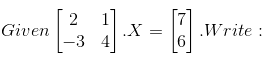(i) The order of the matrix X.

(ii) The matrix X.

Solution:

(i) Let the order of the matrix be a x b.

Then, we know that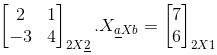Thus, for multiplication of matrices to be possible

a = 2

And, form noticing the order of the resultant matrix

b = 1

(ii)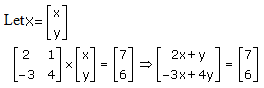On comparing the corresponding terms, we have

2x + y = 7 and

-3x + 4y = 6

Solving the above two equations, we have

x = 2 and y = 3

Thus, the matrix X is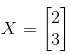5. Evaluate: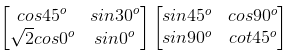Solution: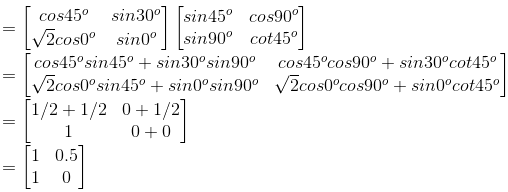6.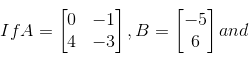3A x M = 2B; find matrix M.

Solution:

Given,

3A x M = 2B

And let the order of the matric of M be (a x b)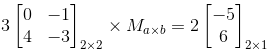Now, it’s clearly seen that

a = 2 and b = 1

So, the order of the matrix M is (2 x 1)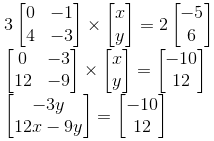Now, on comparing with corresponding elements we have

-3y = -10 and 12x – 9y = 12

y = 10/3 and 12x – 9(10/3) = 12

12x – 30 = 12

12x = 42

x = 42/12 = 7/2

Therefore,

Matrix M =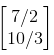7.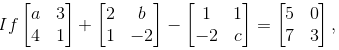find the values of a, b and c.

Solution: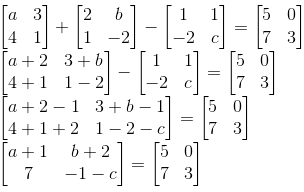On comparing the corresponding elements, we have

a + 1 = 5 ⇒ a = 4

b + 2 = 0 ⇒ b = -2

-1 – c = 3 ⇒ c = -4

8.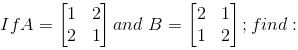(i) A (BA) (ii) (AB) B.

Solution:

(i) A (BA)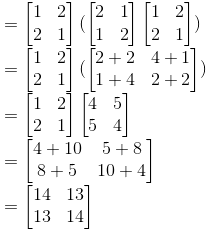(ii) (AB) B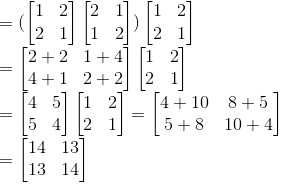9. Find x and y, if: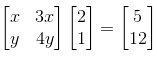Solution: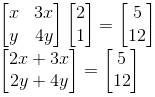Thus, on comparing the corresponding terms, we have

2x + 3x = 5 and 2y + 4y = 12

5x = 5 and 6y = 12

x = 1 and y = 2

10. If matrix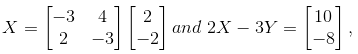find the matrix ‘X’ and matrix ‘Y’.

Solution: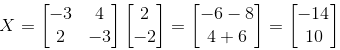Now,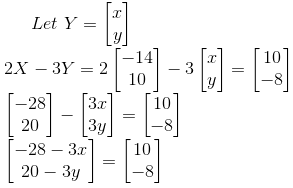On comparing with the corresponding terms, we have

-28 – 3x = 10

3x = -38

x = -38/3

And,

20 – 3y = -8

3y = 28

y = 28/3

Therefore,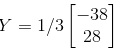11. Given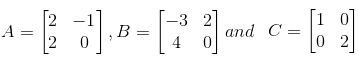find the matrix X such that:

A + X = 2B + C

Solution: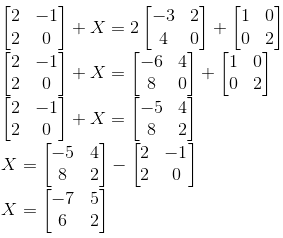12. Find the value of x, given that A2 = B,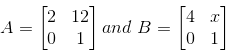Solution: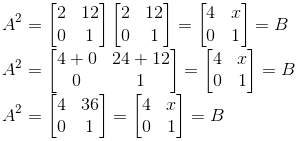Thus, on comparing the terms we get x = 36.